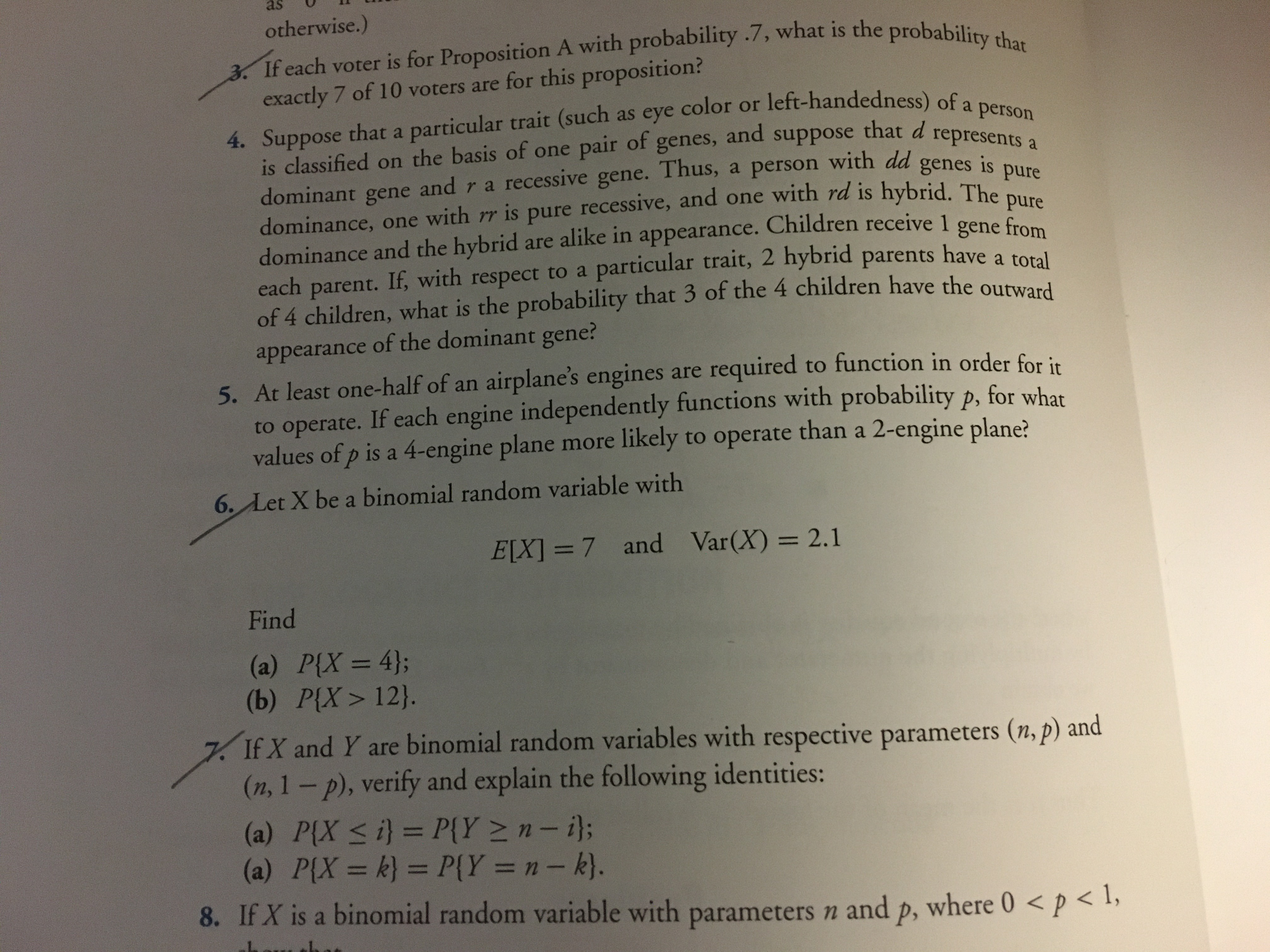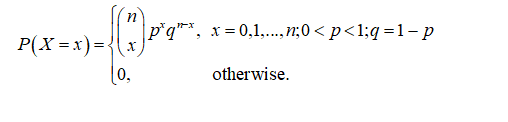# asotherwise.)3 If each voter is for Proposition A with probability .7, what is the probability thatexactly 7 of 10 voters are for this proposition?left-handedness) of a personofgenes,andsuppose that d representeis classified on the basis of one pairdominant gene and r a recessive gene. Thus, a person with dddominance, one with rr is pure recessive, and one with rd is hybrid. Thedominance and the hybrid are alike in appearance. Children receive 1 genc feeach parent. If, with respect to a particular trait, 2 hybrid parents have a toralof 4 children, what is the probability that 3 of the 4 children have the outwardgenes ispurepureappearance of the dominant gene?5. At least one-half of an airplane's engines are required to function in order for irto operate. If each engine independently functions with probability p, for wharvalues of p is a 4-engine plane more likely to operate than a 2-engine plane?6. Let X be a binomial random variable withE[X] =7__and Var(X) = 2.1Find(a) P{X = 4};(b) P{X> 12}.%3D7. If X and Y are binomial random variables with respective parameters (n, p) and(n, 1- p), verify and explain the following identities:(a) P{X < i} = P{Y >n- i};(a) P{X = k} = P{Y = n- k).8. If X is a binomial random variable with parameters n%3D%3D%3D%3DandP,where 0 < p < l,

Question
1 views

Question 3help_outlineImage Transcriptioncloseas otherwise.) 3 If each voter is for Proposition A with probability .7, what is the probability that exactly 7 of 10 voters are for this proposition? left-handedness) of a person of genes, and suppose that d represente is classified on the basis of one pair dominant gene and r a recessive gene. Thus, a person with dd dominance, one with rr is pure recessive, and one with rd is hybrid. The dominance and the hybrid are alike in appearance. Children receive 1 genc fe each parent. If, with respect to a particular trait, 2 hybrid parents have a toral of 4 children, what is the probability that 3 of the 4 children have the outward genes is pure pure appearance of the dominant gene? 5. At least one-half of an airplane's engines are required to function in order for ir to operate. If each engine independently functions with probability p, for whar values of p is a 4-engine plane more likely to operate than a 2-engine plane? 6. Let X be a binomial random variable with E[X] =7__and Var(X) = 2.1 Find (a) P{X = 4}; (b) P{X> 12}. %3D 7. If X and Y are binomial random variables with respective parameters (n, p) and (n, 1- p), verify and explain the following identities: (a) P{X < i} = P{Y >n- i}; (a) P{X = k} = P{Y = n- k). 8. If X is a binomial random variable with parameters n %3D %3D %3D %3D and P, where 0 < p < l, fullscreen
check_circle

Step 1

Binomial distribution:

The binomial distribution gives the probability of number of successes out of n trials in a series of Bernoulli trials.

The probability mass function (pmf) of a binomial random variable X is given as:...

### Want to see the full answer?

See Solution

#### Want to see this answer and more?

Solutions are written by subject experts who are available 24/7. Questions are typically answered within 1 hour.*

See Solution
*Response times may vary by subject and question.
Tagged in

### Probability